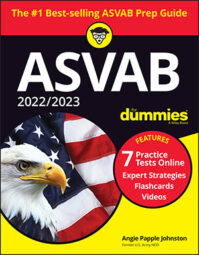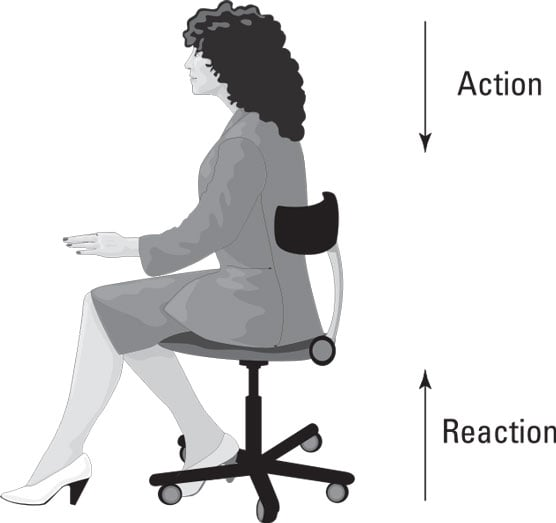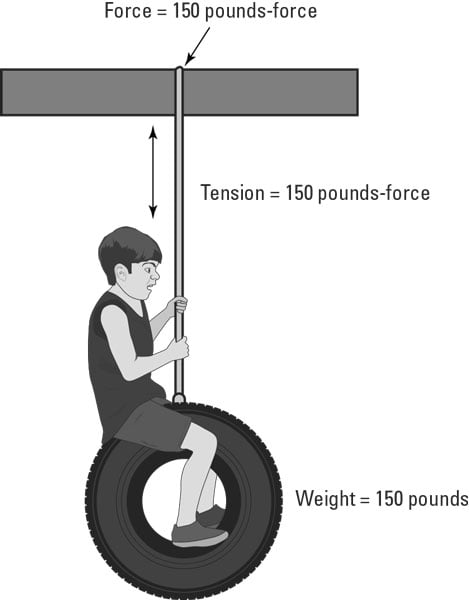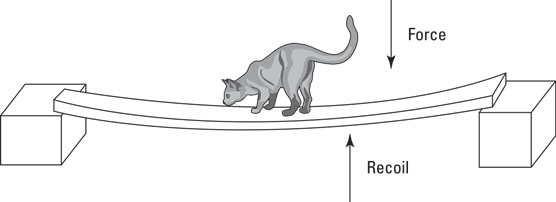##### 2022 / 2023 ASVAB For DummiesYou will want to have a basic understanding of the forces studied in physics for the ASVAB. By applying force (a push or pull), you can open the door or close it, speed it up (slam it) or slow it down (catch it before it slams), or make it change direction (push it shut when the wind blows it open).

In physics, applying force allows changes in the velocity (the speed and direction) of an object. A change in velocity is known as acceleration. Here’s the mathematical formula to determine force:

Force = Mass x Acceleration

Martial artists use this concept all the time. Although a larger fighter may have more size (mass), a smaller fighter can usually speed up more quickly (have more acceleration), possibly resulting in both fighters’ applying the same amount of force. This concept is why 110-pound martial artists can break boards and bricks just as well as 200-pound martial artists.

## The basics of action and reaction

Sir Isaac Newton sure was one of the sharpest crayons in the box. His third law of motion states that for every action (force) in nature, there’s an equal and opposite reaction. In other words, if object A exerts a force on object B, then object B also exerts an equal and opposite force on object A. Notice that the forces are exerted on different objects.An example of action and reaction forces.

As you sit in your chair, your body exerts a downward force on the chair, and the chair exerts an upward force on your body. There are two forces resulting from this interaction: a force on the chair and a force on your body. These two forces are called action and reaction forces.

This force can also be used to describe how a motorboat moves through the water. As the propellers turn, they push the water behind the boat (action). The water reacts by pushing the boat forward (reaction).

## Equilibrium: Finding a balance

Forces are vector quantities. That means they have both a magnitude (size) and a direction associated with them. Forces applied in the same direction as other forces increase the total force, and forces that move in opposite directions reduce the total force. In general, an object can be acted on by several different forces at any one time.

A very basic concept when dealing with forces is the idea of equilibrium or balance. When two or more forces interact so that their combination cancels the other(s) out, a state of equilibrium occurs. In this state, the velocity of an object doesn’t change. The forces are considered to be balanced if the rightward forces are balanced by the leftward forces and the upward forces are balanced by the downward forces.

If an object is at rest and is in a state of equilibrium, then it’s at static equilibrium. Static means being stationary or at rest. For example, a glass of water sitting on a table is at static equilibrium. The table exerts an upward force on the glass to counteract the force of gravity.

## Under pressure: Spreading out the force

Pressure is a measurement of force over an area. Pressure is usually measured in pounds per square inch (psi). The formula for deriving pressure is

If 50 pounds of force is exerted on 10 square inches of surface, the amount of pressure is 5 pounds per square inch (5 = 50 / 10).

Consider this: If you’re sleeping in bed, the amount of pressure being exerted per square inch is much less than when you’re standing on your feet. The surface area of the bottoms of your feet (supporting all that weight) is much less than the surface area of all your body parts that touch the mattress.

Ever wonder how a person can lie on a bed of nails? The answer involves elementary physics. His or her body rests evenly on hundreds of nails; therefore, no individual nail exerts a great amount of pressure against the skin. Have you ever seen someone stand on a bed of nails? It’s unlikely because more pressure is on the feet, and the nails would puncture the feet.

A barometer is a gauge that measures atmospheric pressure. Normal atmospheric pressure is 14.7 psi. A change in air pressure means the weather is about to change.

## Kinds of forces

Here are some of the forces that act on objects:
• Friction: Resistance to the motion of two objects or surfaces that touch
• Gravity: The physical property that draws objects toward the center of Earth (and other objects that have mass)
• Magnetism: The property of attracting iron or steel
• Recoil: The property of kicking back when released
• Static electricity: The production of stationary electrical charges, often the result of friction

### Friction: Resisting the urge to move

When one surface (such as a floor) resists the movement of another surface (the bottom of a piano), the result is frictional resistance. (This friction isn’t like resisting orders to cut the grass. That type of resistance may cause friction between you and your dad, but I’m talking about a different kind of resistance here.)

In order to perform work — that is, to get an object to move in the direction you’re pushing or pulling — sometimes you have to overcome friction by applying more force. For example, when you’re moving a piano across a smooth, vinyl floor, little friction is produced, so the amount of force required to push the piano comes from the piano’s weight and the very minor friction produced by the smooth floor. But when you’re moving a piano across a carpeted floor, more friction is produced, so you have to push harder to move the same piano the same distance.

Rolling friction (like the friction that occurs when you roll a wheel along the pavement) is always less than sliding friction (which occurs when you shove a piano along the floor). If you put wheels on a piano, it’s much easier to push!

You can decrease friction by using a lubricant. Oil, grease, and similar materials reduce friction between two surfaces. So theoretically, if you oil the bottom of a piano, it’s easier to move! (Oiling the bottom of your piano isn’t recommended — for reasons involving the appearance of your floor and piano.)

### Gravity: What goes up must come down

Sir Isaac Newton invented gravity in 1687 when he failed to pay attention while sitting under a tree and got bonked on the noggin by an apple. Before that, gravity didn’t exist, and everyone just floated around. Okay, I’m kidding. Isaac Newton didn’t invent gravity. But the famous mathematician was the first to study gravity seriously, and he came up with the theory (now a scientific law) of how gravity works.

Newton’s law of universal gravitation states that every object in the universe attracts every other object in the universe. Earth produces gravity, and so do the sun, other planets, your car, your house, and your body. The amount (force) of the attraction depends on the following:

• Mass: The force of gravity depends on the mass of (amount of matter in) the object. If you’re sitting in front of your television, you may be surprised to know that the television set is attracting you. However, because the mass of the TV is so small compared to the mass of Earth, you don’t notice the physical “pull” toward the television set.

Note that the force of gravity acting on an object is equal to the weight of the object. Of course, other planets have lesser or greater masses than Earth, so the weight of objects on those planets will be different.

• Distance: Newton’s law also says that the greater the distance is between two objects, the less the objects will attract each other. In other words, the farther away an object is from Earth (or any large body), the less it will weigh. If you stand at the top of a high mountain, you will weigh less than you will at sea level. Don’t get too excited about this weight-loss technique. Gravitational pull isn’t the next big diet craze. The difference is incredibly small. Sorry!

For an object to really lose weight, it must be far away from Earth (or any other large body). When an object is far enough away from these bodies that it experiences practically no gravitational pull from them, it’s said to experience weightlessness — just like the astronauts you see on TV.

Gravity pulls objects downward toward the center of Earth, so the old saying “what goes up must come down” is appropriate when discussing gravity. If you fire a bullet straight up into the air, it will travel (overcoming the force of gravity) until it reaches its farthest or highest point, and then it will fall.

### Applying force to two ends: Tension

Tension force is the force transmitted through a rope, string, or wire when force is applied to both ends. The force is the amount of tension directed along the rope, string, or wire and pulls equally on the objects at both ends. Tension force is usually measured in either pounds-force or newtons (N); 4.45 newtons equal 1 pound-force.An example of tension force.

### Elastic recoil: The trampoline of physics

Liquids and gases don’t have a specific shape, but solid matter does. Solids are perfectly happy with the way they look and resist changes in shape. If you exert a force on a solid shape, it responds by exerting a force in the opposite direction. This force is called elastic recoil.

Take a look at the following figure. The cat is standing on a board suspended on two blocks. While the board bends, the cat can feel the force of the board trying to regain its original shape. If the cat steps off the board, the board will spring back to its normal state.The concept of elastic recoil.